# How to Use MQ-4 Methane Gas Sensor

The MQ-4 is one of many gas sensors ready to be interfaced with microcontrollers. Just like the rest of the MQ sensors, the MQ-4 is most sensitive to a particular gas. This time, it’s methane, although the sensor can still detect other flammable gases like butane and propane.

### MQ-4 Methane Sensor Overview

At the heart of the MQ-4 is a heater and electrochemical sensor. When the target gas enters the membrane and reaches the sensor, it undergoes a redox reaction which creates current. This current is stronger for sensors at specific gases. In the case of the MQ4, it’s more sensitive to methane, butane and propane.

If you are looking to buy a MQ-4 sensor, you should choose the one that comes in a breakout board like this:

This breakout board has four output pins, namely A0, D0, GND and VCC.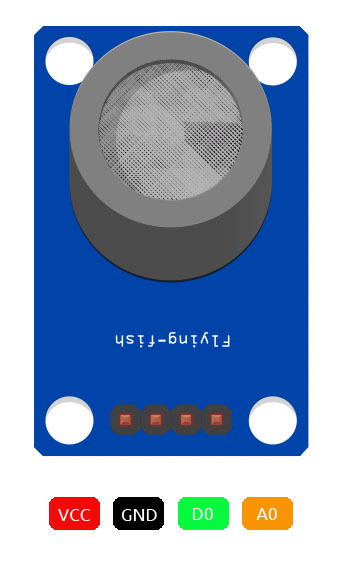The power pins VCC and GND can be connected directly to an Arduino’s 5 V pin and GND respectively.

### Using Digital Output

The D0 pin generates a high (equal to VCC) when in the presence of methane gas and low (equal to around 0.1 V) otherwise. You can calibrate this “digital” output through the trimmer pot on the board.

If your project only requires detection of methane then reading the D0 pin will do. Here’s a circuit diagram with an Arduino UNO:Here, the D0 pin connects to digital pin 2 of the Arduino. The Arduino sketch below uses an interrupt so that the microcontroller always detects the MQ-4 first.

```/* MQ4 Sensor - Digital Output Example
* by R. Pelayo
*
* From TeachMeMicro (www.teachmemicro.com/arduino-mq4-methane-sensor
*
* Date Created: 09/11/2020
*/
const byte MQ4_Pin = 2; //MQ4 D0 pin

void setup() {
pinMode(MQ4_Pin, INPUT_PULLUP);
attachInterrupt(digitalPinToInterrupt(MQ4_Pin), sensor_triggered, CHANGE); //attach interrupt on MQ4 pin
Serial.begin(9600);
}

void loop() {
// Do anything you want here
}

void sensor_triggered() {
Serial.println("Methane detected!"); // Output to serial monitor
}```

If however you need to determine methane concentration, you’ll need the A0 pin.

### Using Analog Output

#### Determining PPM Equation

The sensitivity curve of the MQ-4 is shown below: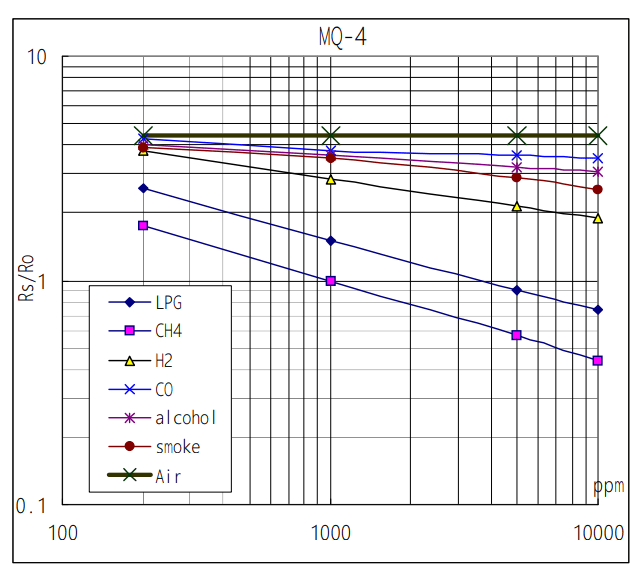This curve is from the device datasheet and shows the sensitivity of the MQ-4 to gases. As seen here, it’s most sensitive to CH4, the chemical name of methane. Absent in the curve are propane and butane gases although both are known components of LPG (which is second to methane in this curve).

The curve is a log-log scale and shows the relationship between RS/R0 and gas concentration in parts-per-million (PPM). RS/R0 is the ratio of sensor resistance at target gas (RS) and resistance in clean air (R0). Hence, by knowing RS/R0, we can determine the concentration of the gas in PPM.

We take two points on this graph to derive a formula. This formula will then be used in our Arduino sketch later on.

The most obvious point is when RS/R0 = 1 and PPM = 1000. The second point is when RS/R0 is somewhere around 0.58 and PPM = 5000. The equation starts with:Here, we will assign Y1 = 1, X1 = 1000 from the first point and Y2 = 0.58 and X2 = 5000 from the second point. Substituting these values in the equation above: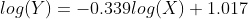Changing Y to RS/R0 and X to PPM and solving for PPM:We can now use this formula in our sketch. But before that, we need to determine the resistance ratio RS/R0.

#### Methane PPM Output Arduino Sketch

As mentioned, RS is the sensor resistance in the presence of Methane while R0 is the sensor resistance in clean air. Of these two, R0 would be easier to determine. We measure the resistance of the electrodes 1-6 or 4-3 (see figure below) using an ohmmeter.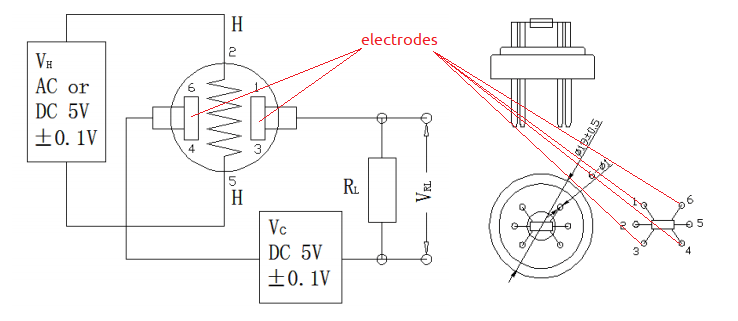My MQ-4 electrodes give out 945 ohms of resistance for both 1-6 and 4-3 electrodes. This means my R0 is 945 ohms.

The value of RS would have to be known through a sketch. The MQ-4 breakout board uses this schematic: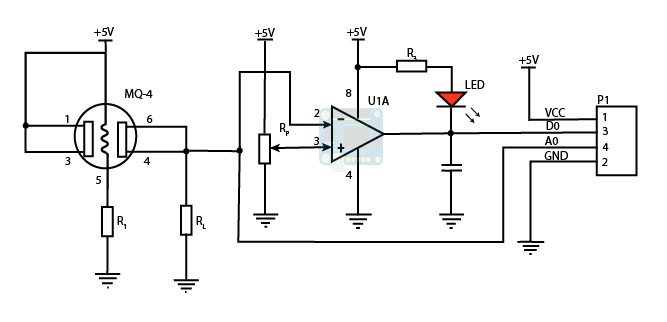As you can see, Aout connects to one of the electrodes and in parallel to a resistor RL. This means the electrode resistance creates a voltage divider with RL and the voltage at Aout is:

Here, RS is our target electrode resistance which varies depending on methane concentration.

BTW, RL is an SMD resistor with label 102. This corresponds to a resistance of 1k.

So to get RS we use this formula:

The Arduino sketch to give out methane concentration in PPM is now:

```/* MQ4 Sensor - Analog Output Example
* Prints out methane concentration in PPM to serial monitor
* by R. Pelayo
*
* From TeachMeMicro (www.teachmemicro.com/arduino-mq4-methane-sensor
*
* Date Created: 09/11/2020
*/
const byte MQ4_Pin = A0; //MQ4 A0 pin
const int R_0 = 945; //Change this to your own R0 measurements

void setup() {
Serial.begin(9600);
}

void loop() {
Serial.println(getMethanePPM());
}

/*
* getMethanePPM returns a float value in PPM of methane concentration
*/
float getMethanePPM(){